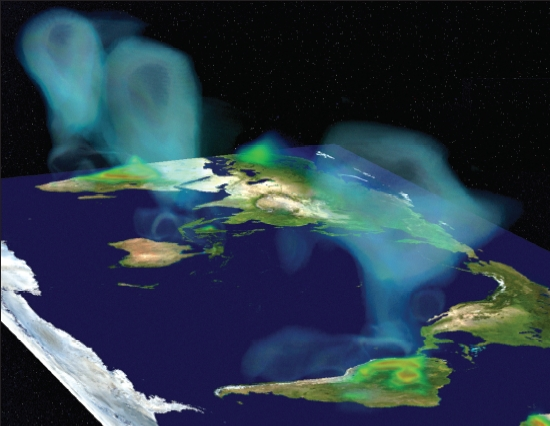Syllabus

This class covers important classes of numerical methods for partial differential equations, notably finite differences and Fourier-based spectral methods. The emphasis is on a solid understanding of the accuracy of these methods, with a view on the role they play in today's science and engineering problems. The class is suitable for graduate students from all departments who have affinities with mathematics.

Topics:

• Short review of PDE (02/01 and 02/03)
• Finite differences for elliptic problems, preconditioning (02/03 to 02/24)
• Finite differences for parabolic and hyperbolic problems, ...
• Stability, consistency, convergence, ...
• Dissipation, dispersion, group velocity (03/01 to 03/17)
• Spectral methods (03/29 to 04/12)
• Finite volume methods for conservation laws (04/14 to 05/05)
• plus a short intro to either finite elements, or boundary integral equations

(Dates are tentative.)

Figures: top-right, simulation of gravitational waves generated by a black hole merger; bottom-left, simulation of turbulent combustion in a jet engine. Credit: SciDAC, Department of Energy.

Reference material

The material will be inspired from various sources.

NEW: typeset notes, 02/14 version.

Handwritten class notes from last year:

Some Matlab scripts used in class last year:

The three most important books are:

• R. LeVeque, Finite difference methods for ordinary and partial differential equations, SIAM, 2007.
• N. Trefethen, Spectral methods in Matlab, SIAM, 2000.
• R. LeVeque, Finite volume methods for hyperbolic problems, Cambridge University Press, 2002.Prerequisites: Some undergraduate familiarity with ordinary differential equations, partial differential equations, Fourier transforms, and linear algebra including solving systems of equations. The assignments will involve basic computer programming in the language of your choice (Matlab recommended).

If you'd like to brush up on your PDE from a not-too-mathematical source, a good book is "Partial differential equations" by W. Strauss.

Figure: simulation of atmospheric CO2 distribution. Credit: SciDAC, Department of Energy.

Who, when, and where

Date and Time: Tu-Th, 1:00-2:30, room 2-131. Instructor: Laurent Demanet. Office hours: W-F, 2:00-4:00, room 2-392.

Evaluation

50% homework, 50% course project.

The homework problem sets will consist of both theoretical problems and numerical experiments. No late copy will be allowed, but the lowest score will be dropped. Collaboration allowed, but the codes and copies you turn in must be original and written by you.

• Problem set 1: due 02/24
• Problem set 2: due 03/17.
• Problem set 3: due 04/14.
• Problem set 4: Implement a finite volume method for the (inviscid) Burgers equation in the case of a transonic rarefaction wave. Please explain why your method chooses the correct entropy solution. Hint: see section 12.3 in LeVeque's book. Due 05/03.

The course project will be of a computational or mathematical nature. Each student will have a different project (hopefully tailored to their taste). The project report should be written like a publication: clear and concise. It is a good idea to use LaTeX for the typesetting. Here is a list of possible projects. Important dates:

• Choose a project by 02/17. Requires consent of the instructor.
• Progress checkup: 03/30 (schedule a meeting with the instructor).
• Project presentations: 05/10 and 05/12.
• Project report due: 05/12.